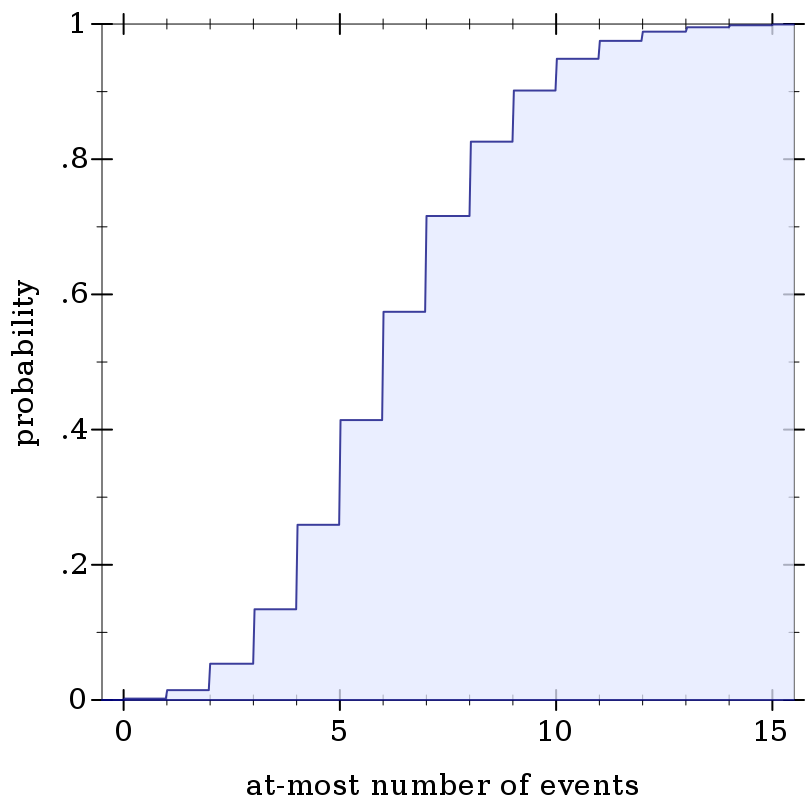#### 9.4Integer Distribution Families

Mathematically, integer distributions are commonly defined in one of two ways: over extended reals, or over extended integers. The most common definitions use the extended reals, so the following distribution object constructors return objects of type Real-Dist.

(Another reason is that the extended integers correspond with the type (U Integer +inf.0 -inf.0). Values of this type have little support in Racket’s library.)

This leaves us with a quandary and two design decisions users should be aware of. The quandary is that, when an integer distribution is defined over the reals, it has a cdf, but no well-defined pdf: the pdf would be zero except at integer points, where it would be undefined.

Unfortunately, an integer distribution without a pdf is nearly useless. In measure-theory parlance, the pdfs are defined with respect to counting measure, while the cdfs are defined with respect to Lebesgue measure. So the pdfs of these integer distributions are pdfs defined over integers, while their cdfs are defined over reals.

Most implementations, such as R’s, make the same design choice. Unlike R’s, this implementation’s pdfs return +nan.0 when given non-integers, for three reasons:
• Their domain of definition is the integers.

• Applying an integer pdf to a non-integer almost certainly indicates a logic error, which is harder to detect when a program returns an apparently sensible value.

• If this design choice turns out to be wrong and we change pdfs to return 0.0, this should affect very few programs. A change from 0.0 to +nan.0 could break many programs.

Integer distributions defined over the extended integers are not out of the question, and may show up in future versions of math/distributions if there is a clear need.

##### 9.4.1Bernoulli Distributions

 syntax
 procedure(bernoulli-dist prob) → Bernoulli-Dist prob : Real
 procedure d : Bernoulli-Dist
Represents the Bernoulli distribution family parameterized by probability of success.

(bernoulli-dist prob) is equivalent to (binomial-dist 1 prob), but operations on it are faster.

Examples:
 > (define d (bernoulli-dist 0.75)) > (map (distribution-pdf d) '(0 1)) '(0.25 0.75) > (map (ordered-dist-cdf d) '(0 1)) '(0.25 1.0) > (define d (binomial-dist 1 0.75)) > (map (distribution-pdf d) '(0 1)) '(0.25 0.75) > (map (ordered-dist-cdf d) '(0 1)) '(0.25 1.0)

##### 9.4.2Binomial Distributions

 syntax
 procedure(binomial-dist count prob) → Binomial-Dist count : Real prob : Real
 procedure d : Binomial-Dist
 procedure d : Binomial-Dist
Represents the binomial distribution family parameterized by count (number of trials) and probability of success.

Examples:
> (define d (binomial-dist 15 0.6))
 > (plot (discrete-histogram (map vector (build-list 16 values) (build-list 16 (distribution-pdf d)))) #:x-label "number of successes" #:y-label "probability")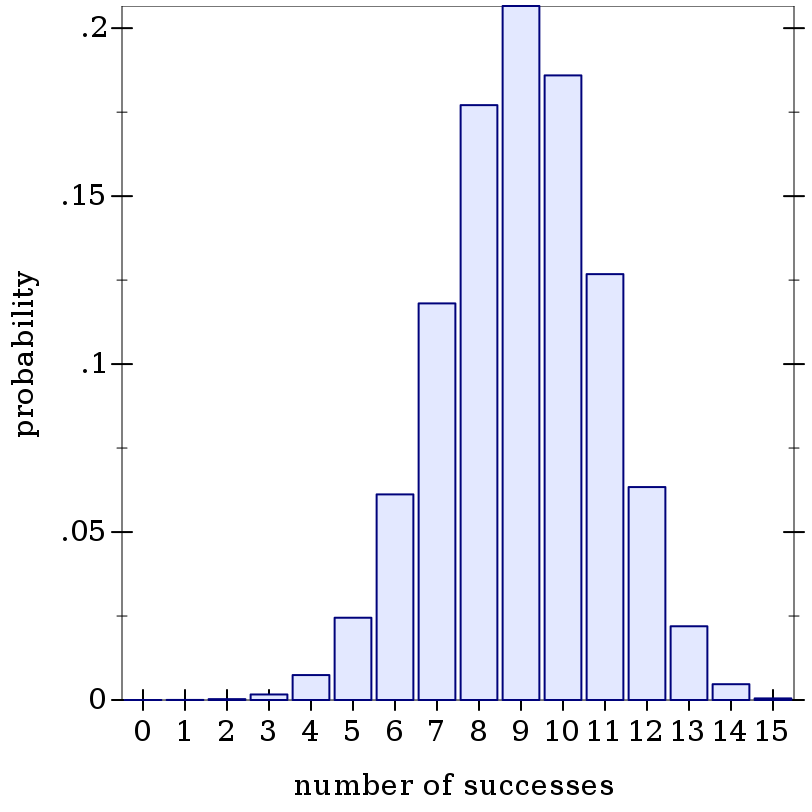> (plot (function-interval (λ (x) 0) (ordered-dist-cdf d) -0.5 15.5) #:x-label "at-most number of successes" #:y-label "probability")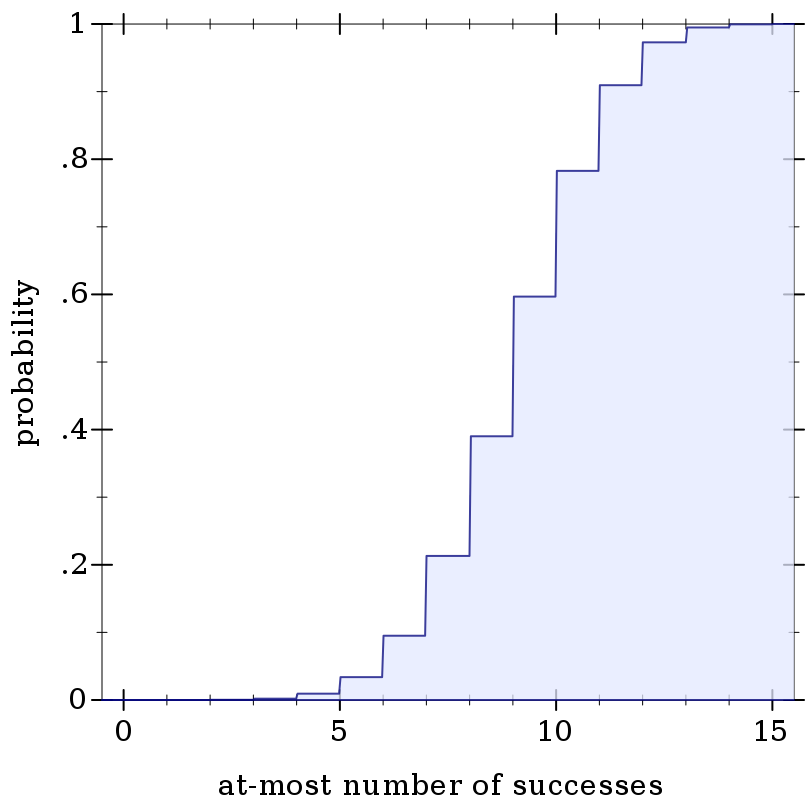##### 9.4.3Geometric Distributions

 syntax
 procedure(geometric-dist prob) → Geometric-Dist prob : Real
 procedure d : Geometric-Dist
Represents the geometric distribution family parameterized by success probability. The random variable is the number of failures before the first success, or equivalently, the index of the first success starting from zero.

Examples:
> (define d (geometric-dist 0.25))
 > (plot (discrete-histogram (map vector (build-list 16 values) (build-list 16 (distribution-pdf d)))) #:x-label "first success index" #:y-label "probability")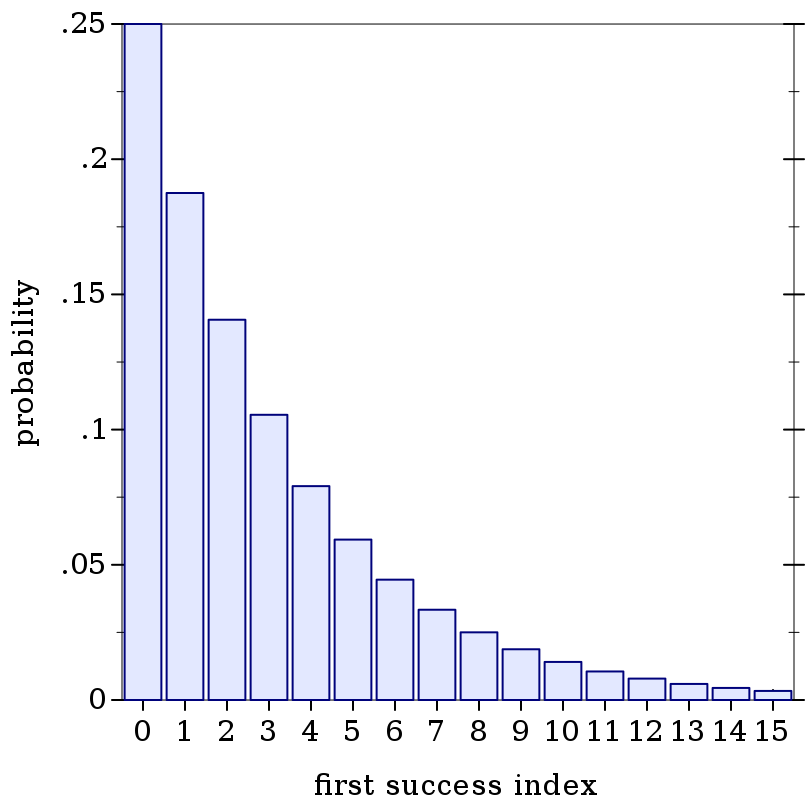> (plot (function-interval (λ (x) 0) (ordered-dist-cdf d) -0.5 15.5) #:x-label "at-most first success index" #:y-label "probability" #:y-max 1)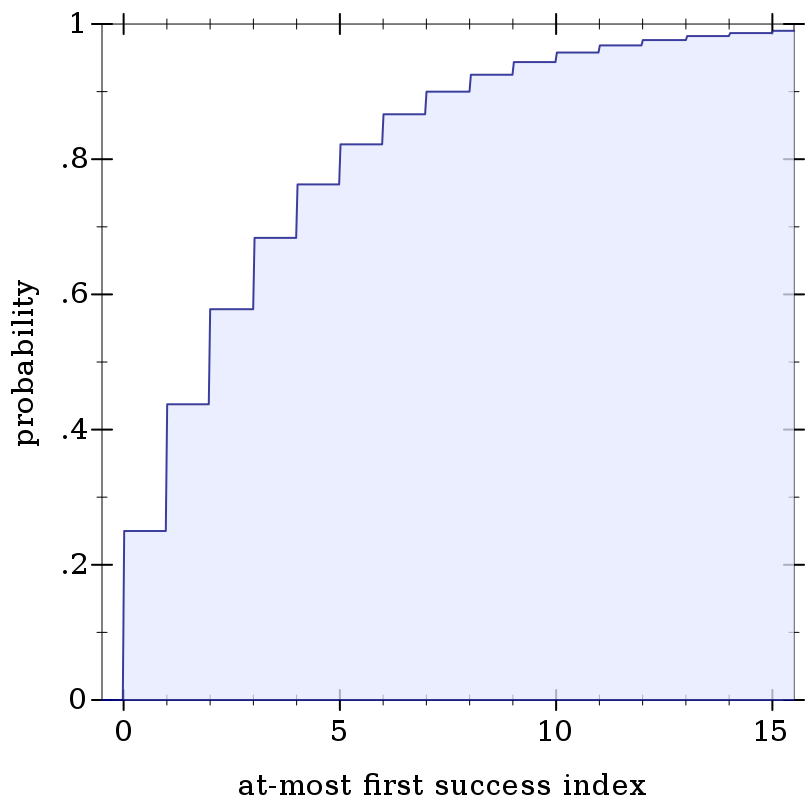##### 9.4.4Poisson Distributions

 syntax
 procedure(poisson-dist mean) → Poisson-Dist mean : Real
 procedure d : Poisson-Dist
Represents the Poisson distribution family parameterized by the mean number of occurrences of independent events.

Examples:
> (define d (poisson-dist 6.2))
 > (plot (discrete-histogram (map vector (build-list 16 values) (build-list 16 (distribution-pdf d)))) #:x-label "number of events" #:y-label "probability")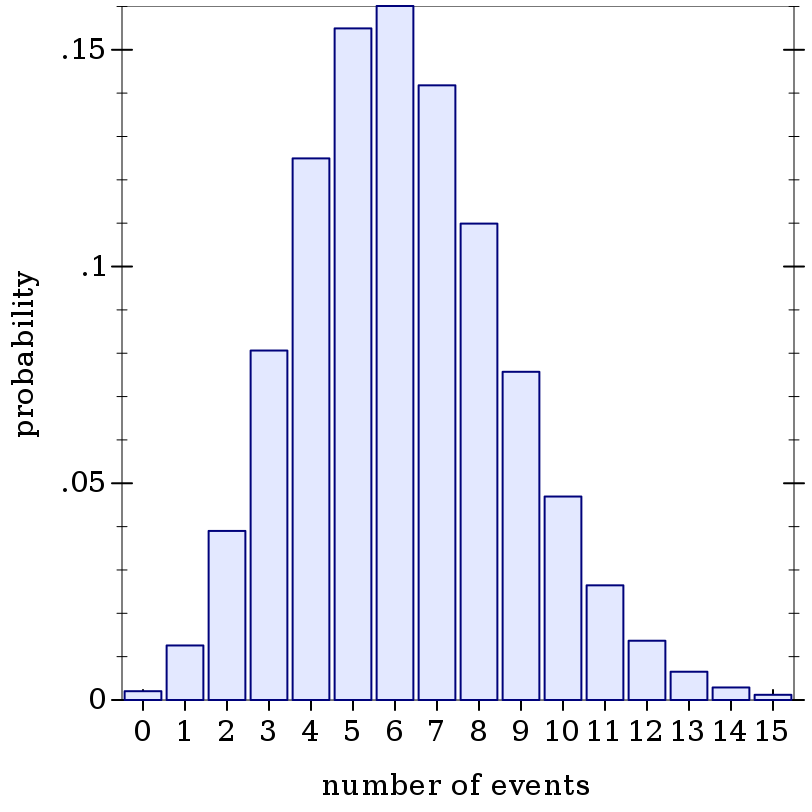> (plot (function-interval (λ (x) 0) (ordered-dist-cdf d) -0.5 15.5) #:x-label "at-most number of events" #:y-label "probability" #:y-max 1)